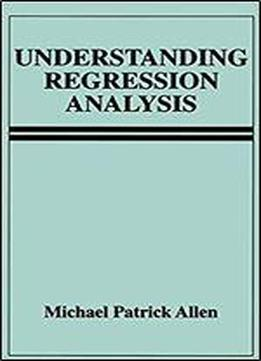Understanding Regression Analysis by Michael Patrick Allen / 1997 / English / PDF

By assuming it is possible to understand regression analysis without fully comprehending all its underlying proofs and theories, this introduction to the widely used statistical technique is accessible to readers who may have only a rudimentary knowledge of mathematics. Chapters discuss: -descriptive statistics using vector notation and the components of a simple regression model -the logic of sampling distributions and simple hypothesis testing -the basic operations of matrix algebra and the properties of the multiple regression model -testing compound hypotheses and the application of the regression model to the analyses of variance and covariance, and -structural equation models and influence statistics.

views: 615